# AP Board 9th Class Maths Solutions Chapter 4 Lines and Angles InText Questions

AP State Syllabus AP Board 9th Class Maths Solutions Chapter 4 Lines and Angles InText Questions and Answers.

## AP State Syllabus 9th Class Maths Solutions 4th Lesson Lines and Angles InText Questions

Question
Observe your surroundings carefully and write any three situations of your daily life where you can observe lines and angles. [Page No. 71]
Solution:
The edges of a blackboard; the edges of a ruler/scale and the edges of a table gives the idea of lines and their corners give the idea of angles.

Question
Draw the pictures in your notebook and collect some pictures. [Page No. 71]
Solution: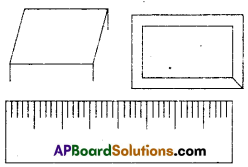Think, Discuss and Write

What is the difference between inter-secting lines and concurrent lines ? [Page No. 74]
Solution:
If the number of lines meeting at a point are only two then they are said to be intersecting lines whereas if the lines are three or more than three meeting at a point then they are said to be concurrent lines.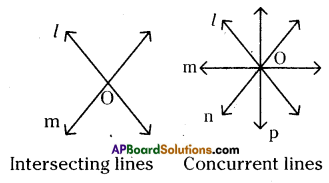Do These

Question 1.
Write the complementary, supplementary and conjugate angles for the following angles, a) 45° b) 75° c) 215° d) 30° e) 60° f) 90° g) 180° [Page No. 76]
Solution: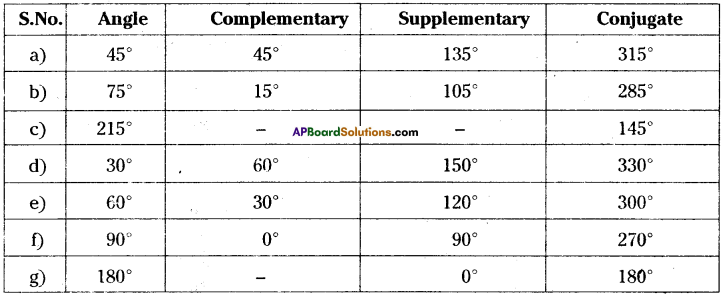Question 2.
Which pairs of the following angles become complementary or supplementary. [Page No. 76]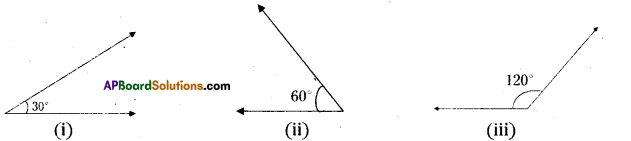Solution:
The angles formed by the figures (i) and (ii) are complementary.
The angles formed by the figures (ii) and (iii) are supplementary.Try This

Question 1.
Find the pairs of adjacent and non- adjacent angles in the given figures. [Page No. 77]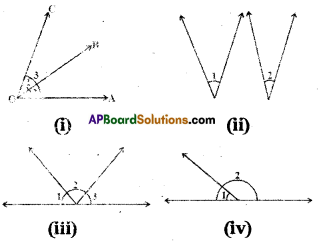Solution:
In figure (i) ∠1 and ∠2 are pair of adjacent angles.
In figure (ii), no adjacent angles.
In figure (iii) (∠1, ∠2), (∠2, ∠3) are pairs of adjacent angles.
In figure (iv) ∠1 and Z2 are adjacent angles.

ii) List the adjacent angles in the given figure. [Page No. 77]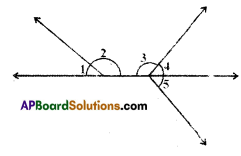Solution:
In the given figure
(∠1, ∠2), (∠3, ∠4), (∠4,∠5) and (∠3, ∠5) are the pairs of adjacent angles.Think Discuss and Write

Question
Linear pair of angles are always supplementary. But supplementary angles need not form a linear pair. Why ? [Page No. 77]
Solution:
A pair of supplementary angles need not necessarily a linear pair because they may exists in separate figures.

Activity

Measure the angles in the following figure and complete the table.
[Page No. 78]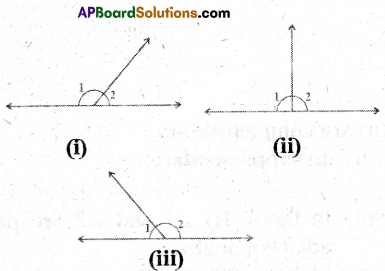Solution: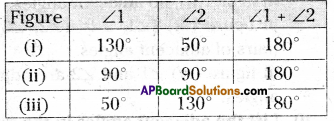Measure the four angles 1,2,3,4 in each of the above figure and complete the table:
[Page No. 79]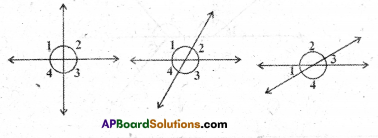Solution: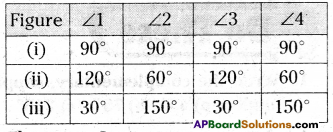Do This

Question 1.
Classify the given angles as pairs of complementary, linear pair, vertically opposite and adjacent angles. [Page No. 80]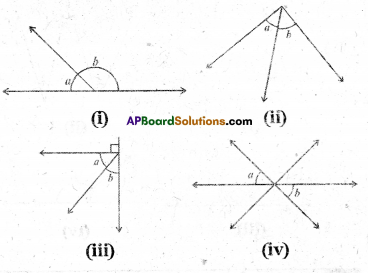Solution:
The pairs of angles a and b in the fig.(i) are linear pair of angles.
The pairs of angles a and b in the fig.(ii) are adjacent angles.
The pairs of angles a and b in the fig.(iii) are complementary angles*
The pairs of angles a and b in the fig.(iv) are vertically opposite angles.

Question 2.
Find the measure of angle ‘a’ in each figure. Give reasons in each case. [Page No. 81]
i)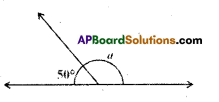a = 180° – 50° = 130°
(linear pair of angles)ii)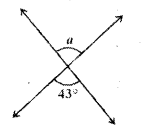a = 43°
( ∵ vertically opposite angles)

iii)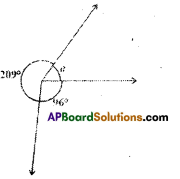a = 360° – (209° + 96°)
= 360° – 305° = 55°
(∵ complete angle = 360°)

iv)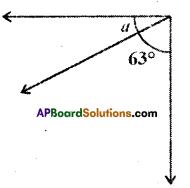a = 90° – 63° = 27°
(pair of complementary angles )Do These

Question 1.
Find the measure of each angle indi-cated in each figure where / and m are parallel lines intersected by a transversal n. [Page No. 87]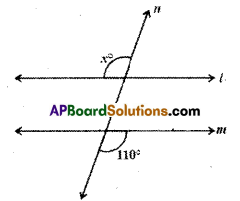Solution:
x = 110° (alternate exterior angles)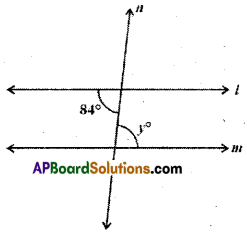Solution:
y = 84° (alternate interior angles)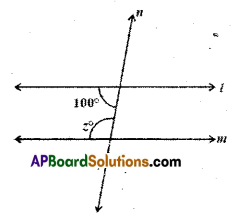Solution:
z = 180° – 100° = 80° .
(interior angles on the same side of transversal)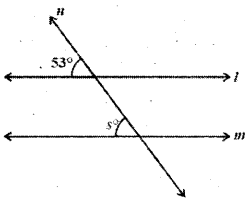Solution:
s° = 53° (pair of corresponding angles)Question 2.
Solve for x and give reasons. [Page No. 88]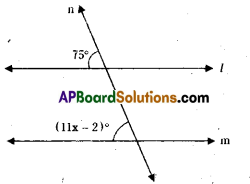11x + 2 = 75°
11x = 75 – 2 = 73
∴ x = $$\frac{73}{11}$$
(∴ pair of corresponding angles are equal).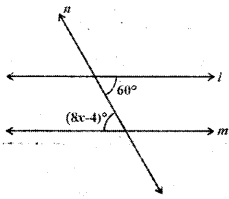Solution:
8x – 4 = 60°
8x = 60 + 4 = 64
∴ x = $$\frac{64}{8}$$ = 8
(∴ alternate interior angles are equal)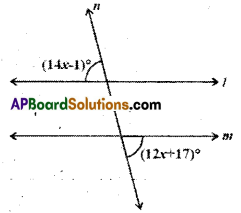Solution:
(14x- 1)°.= (12x + 17)°
14x – 12x = 17 + 1
2x = 18
x = $$\frac{18}{2}$$ = 9
(∴ alternate exterior angles are equal)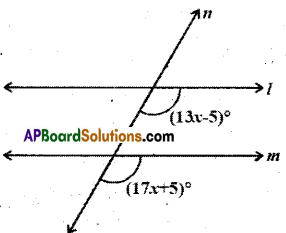Solution:
13x- 5 = 17x + 5
13x- 17x = 5 + 5
– 4x = 10
x = $$\frac{10}{-4}=\frac{-5}{2}$$
(∴ Pair of, corresponding angles are equal).Activity

Question 1.
Take a scale and a ‘set sqaure’. Arrange the set sqare on the scale as shown in figure. Along the slant edge of set sqare draw a line with the pencil. Now slide your set square along its horizontal edge and again draw a line. We observe that the lines are parallel. Why are they parallel ? Think and discuss with your friends. [Page No. 88]
Solution: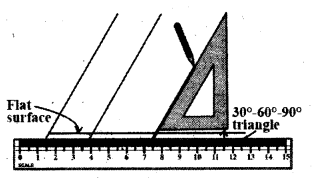A. Student Activity (For Reference .)
All slant lines are parallel making an angle of 60° with the horizontal line. In this figure horizontal line is transversal to the slant line and corresponding angles are equal.

Do This

Question
Draw a line $$\overline{\mathbf{A D}}$$ and mark points B and C on it. At B and C, construct ∠ABQ and ∠BCS equal to each other as shown. Produce QB and SC on the other side of AD to form two lines PQ and RS.

Draw common perpendiculars EF and GH for the two lines PQ and RS. Measure the lengths of EF and GH. What do you observe ? What can you conclude from that ? Recall that if the perpendicular distance between two lines is the same, then they are parallel lines. [Page No. 89]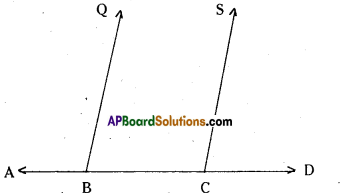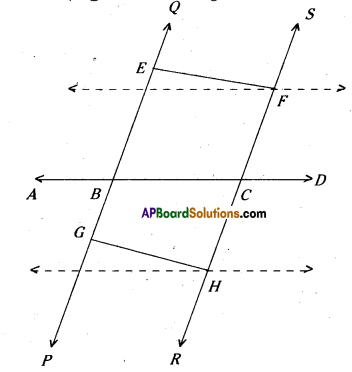Solution:
As ∠ABQ =∠BCS and they lie on the same line AD we can say that BQ // CS. Now EF and GH are the perpendicular distances between two parallel lines PQ and R, we say EF = GH.Try This

Question i)
Find the measure of the question marked angle in the given figure. [Page No. 90]Solution:
? = 70°
[ ∵ from the figure, these two angles are exterior angles on the same side of the transversal]

ii) Find the angles which are equal to ∠P.
Solution:
∠P = ∠Q = ∠R = 110°
(corresponding angles)

Activity

Question
Draw and cut out a large triangle as shown in the figure.
Number the angles and tear them off.
Place the three angles adjacent to each other to form one angle as shown below. [Page No. 97]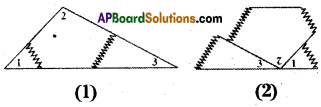1. Identify angle formed by the three adjacent angles ? What is its mea-sure ?
2. Write about the sum of the measures of the angles of a triangle. Now let us prove this statement
using the axioms; and theorems related to parallel lines.
Solution:
Student Activity.Think, Discuss and Write

Question
If the sides of a triangle are produced in order, what will be the sum of exterion angles formed ? [Page No. 99]
Solution:
Let ΔABC and the sides of the triangle is formed by exterior angles.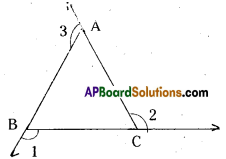∠3 = ∠B + ∠C
∠1 + ∠2 + Z∠3 = 2[∠A + ∠B + ∠C]
= 2 x 180° = 360°
∴ Sum of the exterior angles are 360°.

AP Board 9th Class Maths Solutions